# A New Framework for Recognizing Normal and Epileptic Seizure from Eye Movement Signals Using Genetic Based Convolutional Neural Network

A New Framework for Recognizing Normal and Epileptic Seizure from Eye Movement Signals Using Genetic Based Convolutional Neural Network

Electronics and Communication Engineering, JNTU Ananthapuramu, Andhra Pradesh 515002, India

Electronics and Communication Engineering, VNR Vignana Jyothi Institute of Engineering and Technology, Hyderabad, Telangana 500090, India

Electronics and Communication Engineering, JNTU College of Engineering, Ananthapuramu, Andhra Pradesh 515002, India

Corresponding Author Email:
priyanka_v@vnrvjiet.in
Page:
493-501
|
DOI:
https://doi.org/10.18280/ts.370317
1 February 2020
|
Accepted:
5 May 2020
|
Published:
30 June 2020
| Citation

OPEN ACCESS

Abstract:

The unusual disturbance caused in the neuronal electrical activities inside the brain may lead to epileptic seizures. It is difficult to recognize normal epileptic seizures, as it requires extreme care to identify the epileptic seizure with precise and genuine manner. The existing work did not bridge the gap how accurate the eyeball movement signals can help the detection of epileptic seizure. In this paper, the Electrooculography (EOG) signals are processed to implement a method to predict epileptic seizure. The proposed method epileptic seizure detection using genetic based convolutional neural network (ESD-GCNN) helps in finding the epileptic seizure accurately from the eyeball movement signals. The Normalized Data Nonlinearity (NDN)-LMS is employed in the noise reduction process. During the saccade feature extraction and fixation feature extraction, the proposed method ensures the precision of epileptic seizure detection. The genetic-based CNN helps to make perfect decisions on the existence of epileptic seizure. The proposed research work and the entire research analysis is evaluated in MATLAB environment and the intention is to obtain optimal efficiency for veracity of epileptic seizure detection, compared to current research strategies.

Keywords:

epileptic seizure, feature extraction, genetic algorithm, wiener filter

1. Introduction

Among neurological disorders, epilepsy considered to be a most frequent, that takes place with the rate of 68.8 for every 100,000 persons annually , whereas the age-adjusted rate anticipated to be 44 for every 100,000 persons per year. One-third of epilepsy affected persons remain with seizures despite medication, even though new antiepileptic medicines were introduced in past . Nevertheless, the distress about the seizures' unpredictability and its impacts, diminishes the self-reported value of life, though seizures are under control. The capacity of identifying clinical seizures eases the complexity in handling the treatment-refractory factor of epilepsy . This helping to create perfect seizure records as well as to provide treatments during serious vulnerability of seizure. The medications that expected by rapidly treating seizures can be encouraged by the quick and appropriate identification of seizures as possible . Furthermore benefits procured by the ability of initial detection and prediction before emerging of seizures, which ensures considerable avoidance of mistakes and lessen harm by terminating seizures with the help of appropriate treatments .

World Health Organization (WHO) stated that epilepsy and epilepsy signs identified by the people count of 70 million globally, whereas solely stroke, Alzheimer’s disease, and stroke are listed as most common disorders of the brain. . The routine tasks of the victims have devastated and interrupted due to seizures caused by epilepsy, which may cause extreme risk of premature mortality [7, 8]. Another complicacy in managing epilepsy is a deficiency of neurologists in several countries, in particular, the developing countries are experiencing more of this complicacy. Despite some writings every so often identically mention epilepsy and the seizure, it has to be observed that all seizures are not epileptic and convulsions, besides seizure transpire may because of acute neurological insults (e.g. brain trauma, brain trauma, stroke, and metabolic disturbances), inevitably reflecting a lasting susceptibility to recurrent unprovoked seizures (i.e. epilepsy) [9, 10].

An arise of unexpected anomalous, self-sustained electrical discharge in network structures of cerebral leads to an epileptic seizure (ES), that commonly exists for fewer minutes. The asperity and the period of ES attacks are unable to be determined, besides the prediction of ES is intricate [11, 12], which eventually let the victims and their beloveds harmed and unsecured. So, it necessitates predicting the epileptic attacks in advance, which in turn evading and defeating its unfavourable effects. Epilepsy affected patients' brain activity has been classified into various stages as follows: pre-ictal, ictal, post-ictal, and interictal, which represents the stages as, Immediately Preceding Seizure, during a Seizure, Immediately Following a Seizure, and In-Between Seizures, respectively. This study describes these factors in the following segments in detail. Because of epilepsy's recurrent factor, ES emerges in groups, and seizure troubled patient clusters able to obtain a benefit through the prophesying of further seizures.

2. Related Works

Kher et al.  proposed the approach, that processed the removal of artifacts from EEG signals by using an adaptive filter, which individually employed two inputs for reference namely recorded noisy EEG and clean EEG. For noisy EEG signals, three types of EOG artifacts had recorded (i.e. horizontal eye movement, vertical eye movement, and eye blinks) for five subjects. The process of generating output, to match the reference input, has accomplished by the adaptive filter, on the basis of Least Mean Square (LMS) algorithm.

Selvathi et al.  utilized wavelet transform and Support Vector Machine (SVM) classifier for accomplishing the process of proficiently identifying the seizure existence in EEG signal, by employing the wavelet transform and Support Vector Machine (SVM) classifier. Delta, alpha, theta, beta and gamma sub-bands has derived through segregating the seven levels of EEG signals by using Discrete Wavelet Transform (DWT). Then, by considering the alpha wave to acquire peak amplitude in 100μv range, the seizure has detected.

Finotello et al.  explored the sleep identification issue from EEG data by applying fractal dimension based factors. For deriving the incorporated and beneficial features from EEG signals, the modern approaches have to be elevated utilizing several applications of EEG. The valuable information appended to typical EEG features by the two modern indices accompanying the fractal dimension based features, which in turn enhanced the efficiency of sleep identification.

Park et al.  recommended the novel strategy on the basis of Deep Convolutional Network to identify epileptic seizures. This study befits in Multi-channel EEG signals, besides 1D & 2D Convolutional layer aids in Spatio-Temporal correlation, that is noticed as further feature derived from the detection of an epileptic seizure. 1D & 2D Convolutional layer has employed in each channel's temporal progression of EEG signal and spatial interactions amid EEG signals correspondingly.

Antoniades et al.  utilized deep learning concept for epileptic victims for integration of automated feature generation via Intracranial EEG data with Clinical Insight perspective. The Hierarchical Process is a key role in understanding meaningful features by means of automatic learning. The interpretation of different types of deepest layers in convolved filters is explicated by trained clinicians with the help of Interictal Epileptiform Discharge (IEDs).

Güler and Übeyli  proposed a EEG categorization which is attained utilizing ANFIS along with wavelet coefficients. The integration of adaptive capabilities of the neural network and strategy of fuzzy logic qualitative forms a major role in ANFIS

Guo et al.  accomplished EEG categorization by amalgamating ANN with DWT, RWE. Initially the various frequency bands are obtained from EEG signal by means of DWT. The relative energies which are allied with dissimilar EEG signal frequency bands. Ultimately, classification has performed by the method of feed-forward ANN.

Brignol et al.  suggested an innovative strategy based on phase space, concerning the automatic classification of sleep-wake cycles in human, by utilizing solely two positions of EEG electrode. Nevertheless, in this research, not all the sleep stages have classified through phase space strategy, especially Poincare plot, as it is usually not enforced in linear manner and consumes less time for series analysis.

Brignol et al.  and Abawajy et al.  recommended an inventive multistage strategy in order to categorize huge and extreme dimensional dataset, which integrated the algorithms of Dimensionality Reduction, Multiple Unsupervised Clustering and Several Supervised Classification.

3. Recognizing Normal and Epileptic Seizure from Eye Movement Signals

(NDN)-LMS is employed in the noise reduction process, at the initial stage of this proposed study. Besides, the implementation of saccade feature extraction and fixation feature extraction ensures the precision of epileptic seizure detection, whereas genetic-based CNN helps to make perfect decisions regarding the existence of epileptic seizure. The overall processing flow of the proposed research work ESD-GCNN is shown in the following Figure 1.

## 17.1.png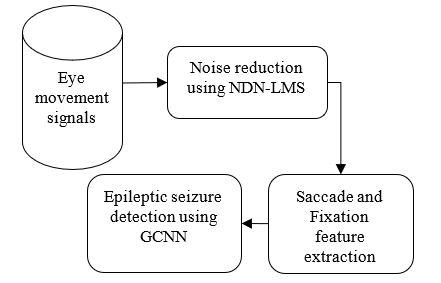Figure 1. Overall processing flow of ESD-GCNN

3.1 Noise reduction using normalized data nonlinearity (NDN)-LMS adaptation method

An inventive algorithm of Least-Mean-Square (LMS) has suggested in this study, for filtering signals, concerning the issue of Adaptive Noise Cancellation (ANC). The squared Euclidean norm minimization involving difference weight vector is a key factor with stability limitation which is demarcated across a posteriori estimation error. The Lagrangian approach helps to resolve this, through proposing to define the nonlinear adaptation rule as to generate distinctive inputs and errors, hence necessitated to generalize the normalized (N)LMS algorithm, and considered as optimal elucidation for constrained optimization delinquent. To articulate these issues ,consider tap-input vector x(n) and the intended response d(n), conclude the tap weight vector w(n+1) which is utilized for squared Euclidean norm minimization, whose change given by §w(n+1)=w(n+1)–w(n) in the tap-weight vector w(n+1) concerning its previous value w(n), subject to the constraint w(n+1)Hx(n)=d(n), where H signifying the Hermitian transpose. This constraint infers the disappearance of posteriori error sequence i.e.

[e[k+1](n) = d(n)- w(k+1)Hx(n)=0, for k=1/n]

The Lagrange multipliers approach has performed with the Lagrangian function, which resolves the optimization problem,

L(w(n+1))=||§w(n+1)||2+Re[λ*e[n+1](n)]  (1)

where, λ* denotes the Lagrange multiplier, therefore the normalized step size is specified by   . The further exciting solution may be obtained by relaxing the latter constraint, due to its extreme restrictive nature in real applications. The constrained optimization problem with respect to cost function is as follows

L(w(n+1)) = ||§w(n+1)||2+Re[λ*§e[n+1](n)]  (2)

where, §e[n+1](n) = e[n+1](n) – e[n+1](n-1). The constancy in the sequence of a posteriori errors has guaranteed by this equilibrium constraint, i.e. the sequence of errors provided by the optimal solution wopt(n+1) in possibly a smoother way, the vector wH(n+1) is fixed to zero value, the partial derivative of (3) has taken prominent to

\begin{aligned} \frac{\partial L(w)(n+1))}{\partial w^{H}(n+1)}=& \frac{\partial \delta w^{H}(n+1) \delta w(n+1)}{\partial w^{H}(n+1)} \\ &+\frac{\partial}{\partial w^{H}(n+1)} \\ & \times R e\left[\lambda * e^{[n+1]}(n)-e^{[n+1]}(n\right.\\ &-1)]=0 \end{aligned}  (3)

Since e[n+1](k)=d(k)-wH(n+1)x(k) for k=n, n-1 and Re[z](z+z*), then

$\frac{\partial L(w)(n+1))}{\partial w^{H}(n+1)}=\delta w(n+1)-\frac{1}{2} \lambda^{*} \delta x(n)=0$  (4)

where,  $\delta x(n)=x(n)-x(n-1)$ denotes the two consecutive input vectors variation. Consequently, the algorithm step is

\begin{aligned} \delta w(n+1)=\frac{1}{2} \lambda^{*} & \delta x(n) \Rightarrow w(n+1) \\=& w(n)+\frac{1}{2} \lambda^{*} \delta x(n) \end{aligned}  (5)

The $\delta x^{H}(n)$ is multiplied on both sides of Eq. (5), therefore the Lagrange multiplier is given as

\begin{aligned} \lambda^{*} &=\frac{2 \delta x^{H}(n) \delta w(n+1)}{\|\delta x(n)\|^{2}} \\=&-\frac{2\left(\delta e^{[n+1]}(n)-\delta e^{[n]}(n)\right)^{*}}{\|\delta x(n)\|^{2}} \end{aligned}  (6)

where, $\delta \mathrm{e}^{[\mathrm{n}]}$(n) = e[n](n) – e[n](n-1) represents the priori error sequence difference [indicated by $\delta e(n)$ crisply], as the numerator on the left-hand side of (7) equals

xH(n) w(n+1) – xH(n-1)w(n+1) – xH(n)w(n)+xH(n-1)w(n)

If the right-hand side of (7) $\delta \mathrm{e}^{[\mathrm{n}]}(n)=0$ has enforced by the equilibrium constraint leads to

$\lambda=\frac{2 \delta e^{[n]}(n)}{\|\delta x(n)\| 2^{*}}$  (7)

Eventually, the pursuing Constrained Stability Update Condition (CS-LMS) have assured by the least of the Lagrangian function

$w(n+1)=w(n)+\frac{\delta x(n) \delta e^{*}(n)}{\|\delta x(n)\|^{2}}$  (8)

The generation of extremely solid weight adaption rule is able to be accomplished through the process of presenting a small positive ε constant contained by the denominator for eliminating arithmetical inconsistencies unexpectedly due to disappearance of small squared norm, and through the velocity of the adaption management by escalating the weight increment with a constant step size. Observe that, the algorithm convergence if it has applied by the equilibrium condition. With regards to, Decorrelation, Blind Source Separation, or Deconvolution Applications, there were numerous learning algorithms have been suggested earlier, when the processing variables' simultaneous change has required by the learning. The algorithms of Stochastic Information Gradient (SIG) has a significant role in errors sequence Shannon’s entropy which may up surged or reduced by employing an estimator focusing on Probability Density Function (PDF) and Parzen Windowing an instantaneous value. Also the variable kernel density estimators is a prime factor involved in single sample-based SIG algorithm generalization achieved by CS-LMS algorithm.

The utilization of ensemble-average learning curves is a general task in the research of adaptive filters' statistical efficiency. The desired clean signal's existence s(n) is the main cause of curves deviation in contrast with the problem of ANC. The step size as µ is represented by the weight-error vector description ε(n)=w0-w(n), hence, the evolution of ε(n) may be quantified as

\begin{aligned} \varepsilon(\mathrm{n}+1)=\varepsilon(\mathrm{n}) &-\mu \delta \mathrm{x}(\mathrm{n}) \\ & \times(\delta \mathrm{s}(\mathrm{n})+\delta \mathrm{v}(\mathrm{n})\\ &\left.-\left(\mathrm{w}_{0}-\varepsilon(\mathrm{n})\right)^{\mathrm{H}} \delta \mathrm{x}(\mathrm{n})\right)^{*} \end{aligned}  (9)

where, $\delta[.](n)=[.](n)-[.](n-1)$ and v(n) signifies the primary signal noise in the d(n). The multiple regression model plays a vital role in determining the weight-error vector value v(n)

$v(n)=w_{0}^{H} x(n)+e_{0}(n)$  (10)

By calling on the direct-averaging approach , the aforementioned calculation takes to

\begin{aligned} \varepsilon_{0}(n+1)=\left(I-\mu R_{\delta x}\right) \varepsilon_{0}(n) & \\-\mu \delta x(n) \delta \hat{e}_{0}^{*}(n) \end{aligned}  (11)

where, $\delta \hat{e}_{0}(\mathrm{n})=\delta \mathrm{e}_{0}(\mathrm{n})+\delta \mathrm{s}(\mathrm{n})$ , and the filtering results in mean-squared error specified by

$J(n)=J_{0}+E\left[|s(n)|^{2}\right]+E\left[\varepsilon_{0}^{H}(n) x(n) x(n)^{H} \varepsilon_{0}(n)\right]$  (12)

where, J0=E[|e0(n)2|] and Jmin=J0+E[|s(n)|2]

The Eq. (12) indicates the stochastic evolution on the natural modes is as follows

$v(n+1)=(I-\mu \wedge) v(n)-\phi(n)$  (13)

The unitary similarity transformation is utilized along with the correlation matrix $R_{\delta x}$ , where $\Lambda=Q^{H} R_{\delta x} Q$ is a diagonal matrix which comprises the Eigen values λk of ,$R_{\delta x}$, is a unitary matrix in which the column elements contains an orthogonal set of eigenvectors and the stochastic force vector is described as $\Phi(n)=\mu Q^{H} \delta x(n) \delta \hat{e}_{0}^{*}(n)$ . The following are the properties of this vector

• The stochastic force vector mean is zero:

$E[\boldsymbol{\Phi}(n)]=0$  (14)

• The stochastic force vector correlation matrix is a diagonal matrix:

$E\left[\boldsymbol{\Phi}(n) \phi^{H}(n)\right]=\mu^{2} \hat{J} \Lambda$  (15)

where, $\hat{J}=2\left(E\left[\left|e_{0}(n)^{2}\right|\right]+E\left[\left|s(n)^{2}\right|\right]-\operatorname{Re}\left\{r_{s}(1)\right\}\right)$

, and rs(1) = E[s*(n+1)s(n)].

These properties help to acquire the initial two moments of the natural nodes which aids in to revealing the evolution of J(n) with time step n, whereas the third term (12) considering the approach of direct-averaging, expressed by

\begin{aligned} J_{e x}(n)=\frac{1}{2} \sum_{k=1}^{L} \lambda_{k} E\left[\left|v_{k}(n)\right|^{2}\right] \\ &+E[t r]\left\{v^{H} Q^{H} \operatorname{Re}\{R(1) Q v\}\right\} \end{aligned}  (16)

where, R(1)=E[x(n+1)xH(n)].

The input signal is supposed to be a weakly correlated signal (R(1)~0),  then, the second term may be bounded  value in the last equality of (17) with the first term (natural evolution), i.e.,

$E\left[t r\left\{y^{H} Q^{H} \operatorname{Re}\{R(I)\} Q y\right\}\right]<=$

$(1 / 2) \sum_{k=1}^{L} \lambda_{k} E\left[\left|v_{k}(n)\right|^{2}\right]$

Therefore

\begin{aligned} J_{e x}(n) \leq \sum_{k=1}^{L} \lambda_{k} E\left[\left|v_{k}(n)\right|^{2}\right] & \\ &=\sum_{k=1}^{L} \lambda_{k}\left(\frac{\mu \hat{J}}{2-\mu \lambda_{k}}\right.\\ &+\left(1-\mu \lambda_{k}\right)^{2 n} \\ &\left.\times\left(\left|v_{k}(0)\right|^{2}-\frac{\mu \hat{J}}{2-\mu \lambda_{k}}\right)\right) \end{aligned}  (17)

where, vk(n) represents for the natural mode v(n) kth component. If the exponential factor is omitted with escalating n

$J_{e x}(\infty) \leq \sum_{k=1}^{L} \lambda_{k}\left(\frac{\mu j}{2-\mu \lambda_{k}}\right) \simeq \frac{1}{2} \mu \hat{J} \operatorname{tr}\left\{R_{\delta x}\right\}$  (18)

The reduction in $J_{e x}(\infty)$ is attained whenever

$J_{e x}(\infty) \simeq \frac{1}{2} \mu \hat{J} \operatorname{tr}\left\{R_{\delta x}\right\} \simeq \mu \hat{J} \operatorname{tr}\{R\} \geq \frac{3}{4} J_{\min }$  (19)

i.e., correlated the desired signal robustly, which also pursues from conventional analysis  that 1) the maximum value of µ balances the trade-off between $J_{e x}(\infty)$  and the average time constant, since

$\tau \simeq \frac{L}{\mu t r\left\{R_{\delta x}\right\}}$  (20)

where, L denotes filter length, and 2) a required stability condition i.e. t 0<µ<2/λk, for all k.

Fixation temporal features

F01: Fixrate

The fixation rate: Fixnum/Recdur, Recdur: total recording duration

F02: FixDurDistrStat

DistrS(·) on durations of fixations: FixDuri, i=1,…, FixNum

S01: SacRate

S02: SacDurDistrStat

DistrS(·) on durations of fixations: SacDuri, i=1,…, SacNum

3.3 Epileptic seizure detection using genetic based convolutional neural network

Mapping the network from the field of Artificial Neural Networks Literature to Genetic Algorithm Literature is a great confront, in the utilization of Genetic Algorithm for practising a Deep Convolutional Neural Network, which indicates that the way of employing GA to equip a network. The original instincts preferred that the networks be supposed to perform as members of a population intended for our algorithm, besides the performance of network be supposed to signify the fitness of network, and the iterative process of the algorithm be supposed to "evolve" the population of networks as regards reliable accuracies. Among the confront, the issue of encoding is an initial stage of the challenge, that means, the way of map a network to a chromosome. Each trainable parameters in a composition of the network can be fragmented by conventional paradigms of encoding, then summarize them as an array construct in order to demonstrate the chromosome in the network, that enables every element of the chromosome array carries solely a single parameter of network. For example, encoding the simplest MLP for the MNSIT classification trial (2 hidden layers each holding 512 neurons, 28 × 28 = 784 input neurons and 10 output neurons, leading to a total of 668672 trainable parameters) leads to chromosome with the size of 668672 elements, each carrying one of the trainable parameters. This demonstration fairly performs until the network is diminutive, but present networks are not diminutive in size. Novel systems of CNN are capable of carrying millions of trainable parameters. A classical encoding of these huge networks causes inefficient composition of the chromosome, as it is too huge to manage. Whereas, a chromosome with lesser size ensures the advantages from high speed, and confidently more influential genetic operators. An encoding is needed to administrate huge sized networks that are able to compress the high quantity of trainable parameters as possible to assemble within appropriate size. Chromosome's each component carries either a layer full of its neuron’s ingoing connection weight value or the overall convolution filter accompanying all of its values. Minimization of the networks' chromosome representation size has ensured by this novel encoding paradigm, which enables high-speed operation time, since the enlargement of the network in size. As per our demonstration outcome, this encoding guarantees a faster convergence rate of the development process and ultimate optimum precision in the threshold.

The processing flow of genetic based convolutional neural network is shown in the following Figure 2.

The steps of steady-state scheme for practicing a convolutional neural network as follows:

(1). Initialization: Through this step, Keras aids to initializing networks that have equivalent size as pop_size, by their convolution filter and then designating connection weight values with a random number derived through a truncated normal distribution centred on zero by the built-in Glorot normal initializer of Keras.

(2). Evaluation: During this step, on the basis of each network's accuracy provided by Keras’ model.evaluate() function, the efficiency of every network has been assessed. This specific step has processed in a concurrent manner aided by multi-process archive from Python, which enables the high-speed run time of a program.

(3). Fitness Assignment: On the basis of each network's assessment, it is designated a fitness value of fi, in which the accuracy of the corresponding network has applied.

(4). Selection: Through this step, each network has designated by a probability of selection. A method called Fitness Proportional Selection has applied in this work, which is one among the most familiar selection approaches, also known as Roulette Wheel selection. Through this process, numerous probable needs to be chosen as a parent for reproduction which in turn the network fitness level is elevated. Hence, for the process of reproduction, the probability of each network has been chosen as a parent, expressed by,

$P_{i}=\frac{f_{i}}{\sum_{i=0}^{p o p_{-} s i z e} f_{i}}$  (21)

(5). Crossover: During this step, a new child has engendered by two parents with a few of their characteristics. It is not considered to take fully folded chromosome for this function, rather a semi-folded chromosome composition, that is each of its components are either the convolution filters or the whole ingoing weights of neuron in the entirely inter-linked part. To make it simpler, through extending the components of the layer in a fully folded chromosome, the composition of the semi-folded chromosome has derived. As for this function, the process picks one of the parents on a random basis, then replicates the respective convolution filter from the chosen parent into the child, which has been processed for every convolution filter in the network of the child. As same as the earlier process, one of the parents has been picked randomly, then replicates the overall ingoing weights of the identical neuron from the parent into ingoing weights of the child's intended neuron.We can easily deduce the fact that $\sum_{i=0}^{p o p_{-} \operatorname{siz} e} P_{i}=1$.

## 17.2.png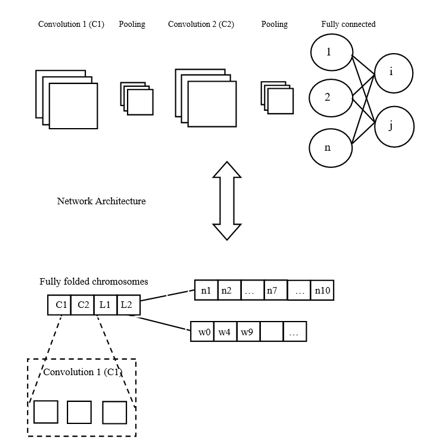Figure 2. Processing flow of genetic based CNN

(6). Mutation: In this step, a new child has engendered by one parent with the majority of their characteristics. The chromosome of the parent has duplicated as accurate as original, and a few elements randomly are chosen from the composition of the semi-folded chromosome. If a convolution filter hold by the chosen element, filter's each value has substituted by Gaussian noise that centred around the value with a 0.5 derivation, whereas if it is a neuron, it has been included to their ingoing weights, a random number derived from the initialization distribution, resulting in engendered a further new child.

(7). Replacement: In order to replace the minimum fit member, the subsequent generation of the population is being fed by the new child, in which process the replacing member detached from the population, besides pop_size remains unchanged all over generations. End of this step indicates a single iteration of the process of steady-state scheme has accomplished.

The aforementioned operation is recurrent from step 2 until some criteria (e.g. number of generations, accuracy, etc.)  is encountered.

Parameter value estimation is the most important step in the CNN classifier which tends to provide the optimal classification outcome. Appropriate selection of parameter values would lead to accurate decision making. In this work, given data set is divided into three subsets for the accuracy and optimal selection of parameter values.

Training set: a set of examples used for learning: to fit the parameters of the classifier g In the MLP case, we would use the training set to find the “optimal” weights with the back-prop rule

Validation set: a set of examples used to tune the parameters of a classifier g In the MLP case, we would use the validation set to find the “optimal” number of hidden units or determine a stopping point for the back propagation algorithm

Test set: a set of examples used only to assess the performance of a fully-trained classifier. In the MLP case, we would use the test to estimate the error rate after we have chosen the final model (MLP size and actual weights). After assessing the final model with the test set, you must not further tune the model

The procedure of parameter selection process is given below:

• Divide the available data into training, validation and test set
• Select architecture and training parameters
• Train the model using the training set
• Evaluate the model using the validation set
• Repeat steps 2 through 4 using different architectures and training parameters
• Select the best model and train it using data from the training and validation set
• Assess this final model using the test set
4. Results and Discussion

The proposed ESD-GCNN has implemented in the MATLAB simulation environment and its performance evaluated in terms of accuracy metrics.

The Figure 3 shows the respective waveform for both the Epileptic Signal and the Normal Signal of the proposed algorithm. It is inferred that there exists noteworthy greater surface area for epileptic seizure EOG signals in contrast with normal EOG signals. The vast EOG signals amplitude of seizure subjects is one of the main factor for up surged surface area involved in the IMFs complex plane of the epileptic seizure EOG signal.

## 17.3.png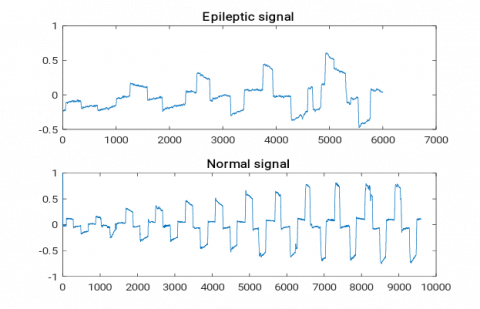Figure 3. Graph for epileptic signal and the normal signal

## 17.4.png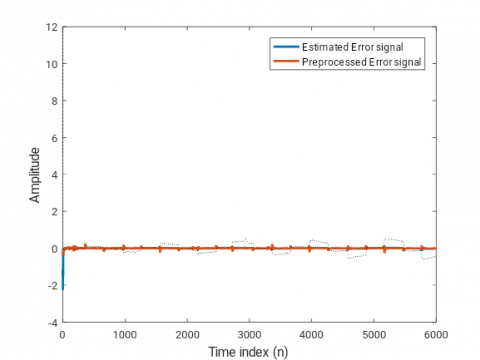Figure 4. Error signal graph

## 17.5.png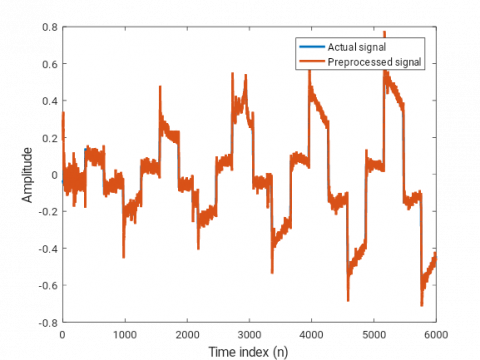Figure 5. Preprocessed signal graph

Figure 4 shows the noise reduction of estimated error signal and the preprocessed error signal of the proposed approach. These signals are generated before applying the proposed noise reduction algorithm.

Figure 5 shows the approach of Normalized Data Nonlinearity (NDN)-LMS employed in the noise reduction process and it shows the graph variation of both the actual signal and the noise reduction signal. The highest peaks clearly shows the variations of the preprocessed signal. The squared Euclidean norm minimization involving difference weight vector is a key factor with stability limitation which is demarcated across a posteriori estimation error.

The Figure 6 shows the MSE comparison graph using normalized data nonlinearity (NDN)-LMS adaptation method. The NDN-LMS learning curve is drawn for up to 600 samples to show the efficiency of the proposed algorithm that how the noises get reduced.

Figure 7 shows the feature extraction graph, where the saccade and fixation features are extracted using the proposed Genetic based CNN approach. In which the implementation of saccade feature extraction and fixation feature extraction ensures the precision of epileptic seizure detection, whereas genetic-based CNN helps to make perfect decisions regarding the existence of epileptic seizure. The ROC graph drawn based on False positive rate versus True positive rate is shown in Figure 8.

## 17.6.png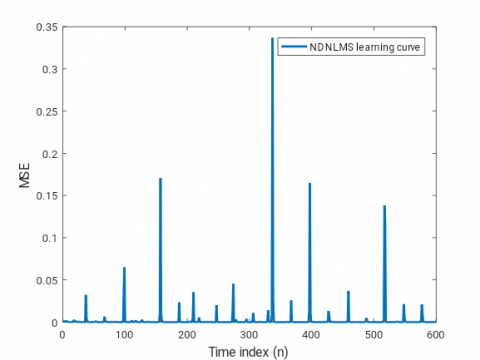Figure 6. MSE comparison graph

## 17.7.png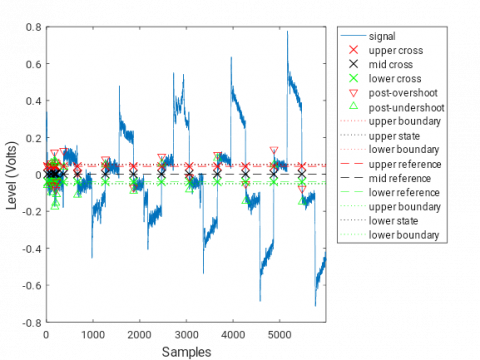Figure 7. Resultant graph for feature extraction

## 17.8.png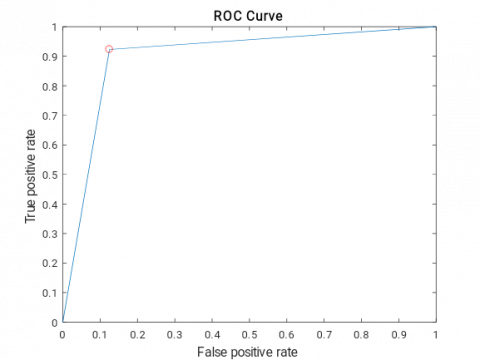Figure 8. ROC curve comparison

The various performance metrics are accuracy, precision, recall and f-measure. The prevailing approaches such as CNN and proposed ESD-GCNN algorithm values are compared for analysis purpose.

Precision: Precision has presented as the proportion of the true positives against the output of true positives as well as false positives, for both imposition and real features. It is different as presented beneath,

Precision $(P)=\frac{T_{p}}{T_{p}+F_{p}}$  (22)

## 17.9.png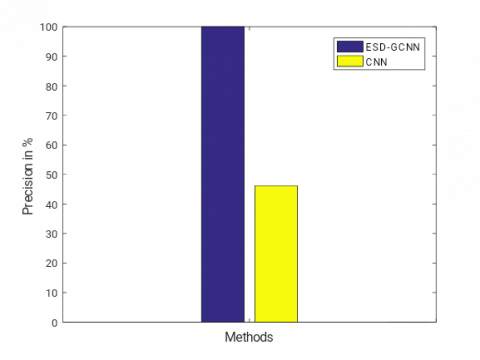Figure 9. Precision comparison

From the above chart, Figure 9 depicts that the suggested approach of ESD-GCNN procures greater precision, compared to existing approaches.

Recall: Recall value relates to the data retrieval value at true positive predict, false negative

$\operatorname{Recall}(R)=\frac{T_{p}}{T_{p}+F_{n}}$  (23)

From the above chart, Figure 10 represents that the suggested approach of ESD-GCNN procures optimum recall. Thus, proposed algorithm of ESD-GCNN is superior than existing algorithm of CNN.

## 17.10.pngFigure 10. Recall comparison

F-measure: It is nothing but the trial accurate assessment.  The score is calculated on the basis of precision p and the recall r of the trial.

$F$ -measure $=2 \cdot \frac{P \cdot R}{P+R}$  (24)

## 17.11.png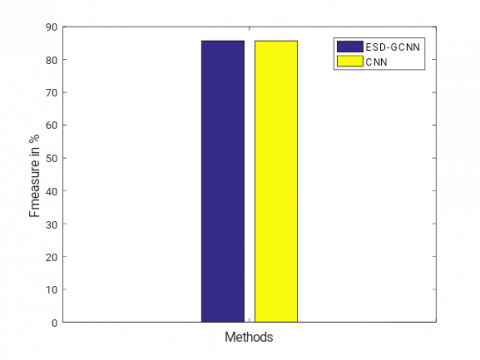Figure 11. F-measure comparison

From the above chart, Figure 11 demonstrates that the suggested approach of ESD-GCNN offers greater f-measure. Thus, the proposed algorithm of ESD-GCNN is superior to the existing algorithm of CNN.

Accuracy: The entire exactness of the system is defined as Accuracy. It is the ratio of total actual classification parameters ( $\left(T_{p}+T_{n}\right)$ with respect to the sum of the classification parameters  $\left(T_{p}+T_{n}+F_{p}+F_{n}\right)$ . The expression of accuracy is as follows

Accuracy $=\frac{T_{p}+T_{n}}{\left(T_{p}+T_{n}+F_{p}+F_{n}\right)}$  (25)

where, TP denotes correct predictions measure for an instance to be negative,

Tn signifies the inappropriate predictions that an instance is positive,

Fp characterizes the incorrect predictions measure that an instance is negative.

And Fn embodies the correct predictions measure for an instance to be positive.

## 12.png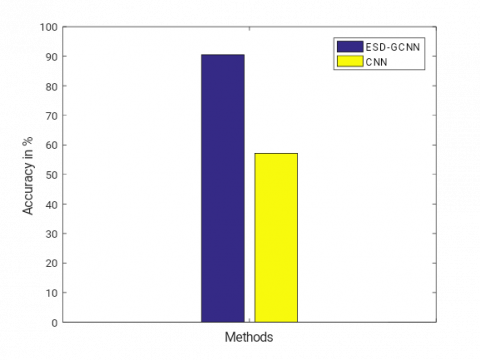Figure 12. Comparison of accuracy metrics

From the above chart, Figure 12 represents that the suggested approach of ESD-GCNN offers optimal accuracy than other approaches.

Table 1. Performance metrics comparison of classifier algorithms in (%)

 Metrics CNN ESD-GCNN Precision 46.15 93.3 Recall 100 100 F-measure 85.7 85.7 Accuracy 57.1429 96
From the analysis given above (Table 1), it is learnt that the proposed ESD-GCNN can ensure the accurate epileptic seizure detection outcome. This is attained in the proposed research work due to introduction of preprocessing technique and the genetic based chromosome optimization. Noise removal ensures to obtain the noise free signal which can be classified more accurately. The genetic algorithm used to select the more optimized parameter values of CNN algorithm. Thus the accurate epileptic seizure recognition can be ensured.
5. Conclusion

The approach of Normalized Data Nonlinearity (NDN)-LMS employed in the noise reduction process, at the initial stage of this proposed study. Besides, the implementation of saccade feature extraction and fixation feature extraction ensures the precision of epileptic seizure detection, whereas genetic-based CNN helps to make perfect decisions regarding the existence of epileptic seizure. Since the entire research analysis evaluated in MATLAB simulation environment, the intention of the proposed approach for delivering optimal efficiency is declared concerning the veracity of epileptic seizure detection, when compared to current research strategies.

References

 Thijs, R.D., Surges, R., O'Brien, T.J., Sander, J.W. (2019). Epilepsy in adults. The Lancet, 55: 475-482. https://doi.org/10.1016/S0140-6736(18)32596-0

 Martin, K., Jackson, C.F., Levy, R.G., Cooper, P.N. (2016). Ketogenic Diet and Other Dietary Treatments for Epilepsy. Cochrane Database of Systematic Reviews, John Wiley & Sons. https://doi.org/10.1002/14651858.CD001903.pub3

 Ratnayake, T.S., Pathirana, P., Melaka, V. (2019). Low cost device to identify Tonic clonic seizure using heartbeat and electromyography. https://www.researchgate.net/project/Low-cost-device-to-identify-Tonic-clonic-seizure-using-heartbeat-and-electromyography.

 Schulze-Bonhage, A. (2017). Brain stimulation as a neuro modulatory epilepsy therapy. Seizure, 44: 169-175. https://doi.org/10.1016/j.seizure.2016.10.026

 Kassiri, H., Tonekaboni, S., Salam, M.T., Soltani, N., Abdelhalim, K., Velazquez, J.L.P., Genov, R. (2017). Closed-loop neurostimulators: A survey and a seizure-predicting design example for intractable epilepsy treatment. IEEE Transactions on Biomedical Circuits and Systems, 11(5): 1026-1040. https://doi.org/10.1109/TBCAS.2017.2694638

 Spiciarich, M.C., von Gaudecker, J.R., Jurasek, L., Clarke, D.F., Burneo, J., Vidaurre, J. (2019). Global health and epilepsy: Update and future directions. Current Neurology and Neuroscience Reports, 19(6): 30. https://doi.org/10.1007/s11910-019-0947-6

 Rasheed, K., Qayyum, A., Qadir, J., Sivathamboo, S., Kwan, P., Kuhlmann, Razi, A. (2020). Machine learning for predicting epileptic seizures using EEG signals: A review. arXiv preprint. arXiv:2002.01925

 Plevin, D., Smith, N. (2019). Assessment and management of depression and anxiety in children and adolescents with epilepsy. Behavioural Neurology. 2019: 1-4. https://doi.org/10.1155/2019/2571368

 Howe, J., Lu, X., Thompson, Z., Peterson, G.W., Losey, T.E. (2016). Intraoperative seizures during craniotomy under general anesthesia. Seizure, 38: 23-25. http://dx.doi.org/10.1016/j.seizure.2016.03.010

 AlQassmi, A., Burneo, J.G., McLachlan, R.S., Mirsattari, S.M. (2016). Benign mesial temporal lobe epilepsy: A clinical cohort and literature review. Epilepsy & Behavior, 65: 60-64. https://doi.org/10.1016/j.yebeh.2016.09.017

 Kovac, S., Kahane, P., Diehl, B. (2016). Seizures induced by direct electrical cortical stimulation-Mechanisms and clinical considerations. Clinical Neurophysiology, 127(1): 31-39. https://doi.org/10.1016/j.clinph.2014.12.009

 Kerr, W.T., Janio, E.A., Braesch, C.T., Le, J.M., Hori, J. M., Patel, A.B. Hwang, E.S. (2017). Identifying psychogenic seizures through comorbidities and medication history. Epilepsia, 58(11): 1852-1860. https://doi.org/10.1111/epi.13888

 Adeli, H., Ghosh-Dastidar, S. (2010). Automated EEG-Based Diagnosis of Neurological Disorders: Inventing the Future of Neurology. CRC Press. https://doi.org/10.1201/9781439815328

 Kher, R., Gandhi, R. (2016). Adaptive filtering based artifact removal from electroencephalogram (EEG) signals. 2016 International Conference on Communication and Signal Processing (ICCSP), Chongqing, China, pp. 0561-0564. https://doi.org/10.1109/ICCSP.2016.7754202

 Selvathi, D., Meera, V.K. (2017). Realization of epileptic seizure detection in EEG signal using wavelet transform and SVM classifier. 2017 International Conference on Signal Processing and Communication (ICSPC), Chennai, India, pp. 18-22. https://doi.org/10.1109/cspc.2017.8305848

 Finotello, F., Scarpa, F., Zanon, M. (2015). EEG signal features extraction based on fractal dimension. 37th Annual International Conference of the IEEE Engineering in Medicine and Biology Society (EMBC), Milano, Italy, pp. 4154-4157. https://doi.org/10.1109/EMBC.2015.7319309·

 Park, C., Choi, G., Kim, J., Kim, S., Kim, T.J., Min, K., Chong, J. (2018). Epileptic seizure detection for multi-channel EEG with deep convolutional neural network. 2018 International Conference on Electronics, Information, and Communication (ICEIC), Honolulu, USA, pp. 1-5. https://doi.org/10.23919/ELINFOCOM.2018.8330671

 Antoniades, A., Spyrou, L., Martin-Lopez, D., Valentin, A., Alarcon, G., Sanei, S., Took, C.C. (2017). Detection of interictal discharges with convolutional neural networks using discrete ordered multichannel intracranial EEG. IEEE Transactions on Neural Systems and Rehabilitation Engineering, 25(12): 2285-2294. https://doi.org/10.1109/TNSRE.2017.2755770

 Güler, I., Übeyli, E.D. (2005). Adaptive neuro-fuzzy inference system for classification of EEG signals using wavelet coefficients. Journal of Neuroscience Methods, 148(2): 113-121. https://doi.org/10.1016/j.jneumeth.2005.04.013

 Guo, L., Rivero, D., Seoane, J.A., Pazos, A. (2009). Classification of EEG signals using relative wavelet energy and artificial neural networks. World Summit on Genetic and Evolutionary Computation (GEC 09), Shanghai, China, pp. 177-183.

 Brignol, A., Al-Ani, T., Drouot, X. (2013). Phase space and power spectral approaches for EEG-based automatic sleep–wake classification in humans: A comparative study using short and standard epoch lengths. Computer Methods and Programs in Biomedicine, 109(3): 227-238. https://doi.org/10.1016/j.cmpb.2012.10.002

 Abawajy, J.H., Kelarev, A.V., Chowdhury, M. (2013). Multistage approach for clustering and classification of ECG data. Computer Methods and Programs in Biomedicine, 112(3): 720-730. https://doi.org/10.1016/j.cmpb.2013.08.002